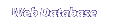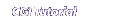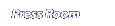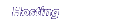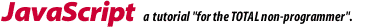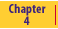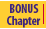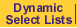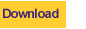Webteacher Software now offers

function encode(text) { Ref="0123456789abcdefghijklmnopqrstuvwxyz.-~ABCDEFGHIJKLMNOPQRSTUVWXYZ"
Result=""
for (Count=0; Count<text.length; Count++) {

Char=text.substring (Count, Count+1);
Num=Ref.indexOf (Char);
EncodeChar=Ref.substring(Num+1, Num+2)
Result += EncodeChar
} document.form1.result.value=Result
}

Looking at the function above, you can see that I have created a loop. The loop uses Count as its counting variable. It will repeat once for each letter in text.

Inside the loop I have written four commands to be executed every time the loop is repeated. Take our initial example of HAL. Here is what would happen during each pass through the loop.

At the start of the loop:
Ref="01234567890abcdefghijklmnopqrstuvwxyz-~ABCDE...." etc.
Result="" (Result is an empty placeholder)
text="HAL"

CommandLoop 1
Count=0
Loop 2
Count=1
Loop3
Count=2
Char=text.substring (Count, Count+1); Char = "H". Char = "A". Char = "L".
Num=Ref.indexOf (Char); Num = 46. Num = 38. Num = 50.
EncodeChar=Ref.substring(Num+1, Num+2) EncodeChar = "I" EncodeChar = "B" EncodeChar = "M"
Result += EncodeChar Result = "I" Result = "IB" Result = "IBM"On to Chapter 5Home | WebData - Web Database Software | Javascript | CGI | Consulting | Map Builder | Contact Us | The Press Room